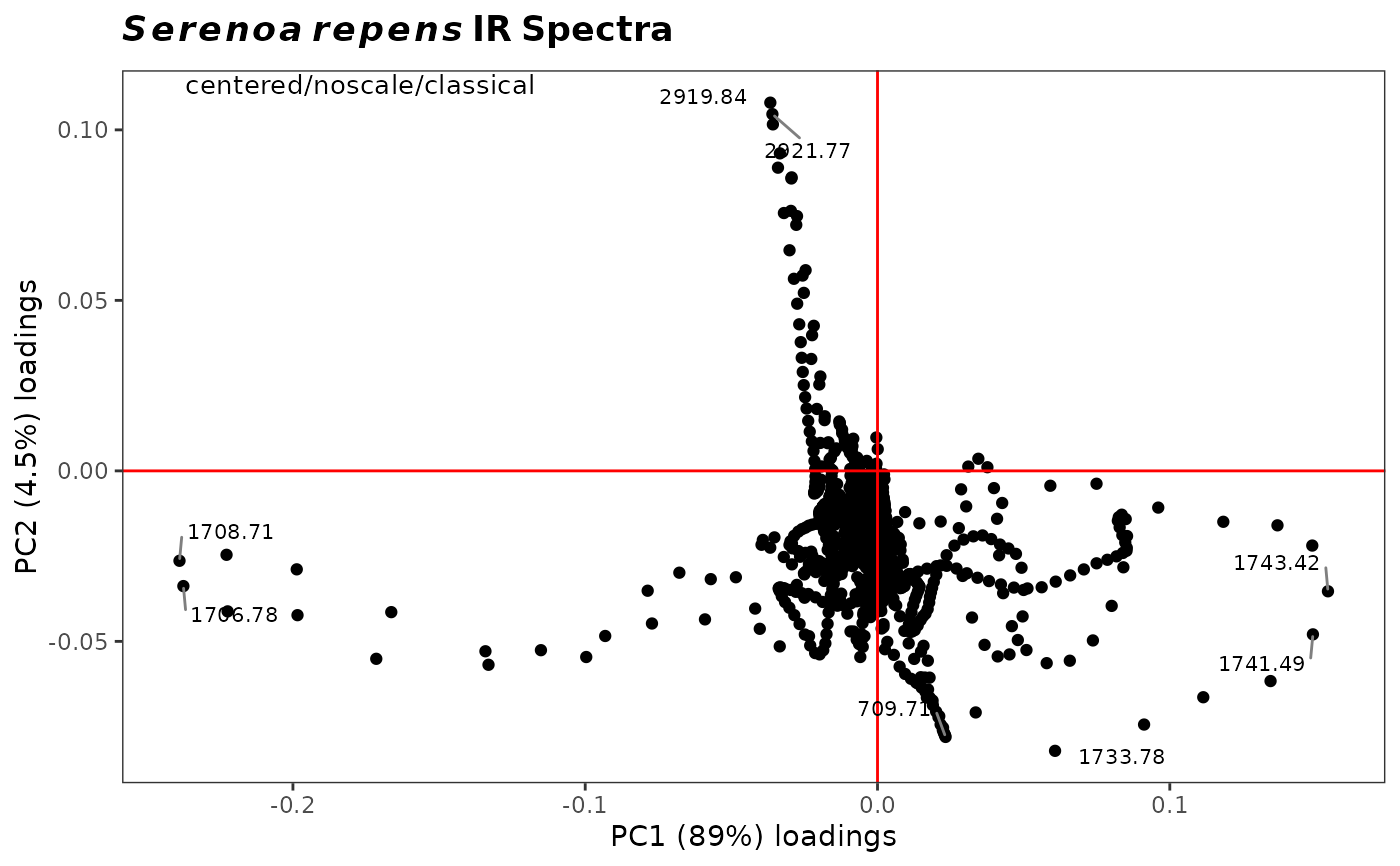Plots two PCA loadings specified by the user, and labels selected (extreme) points. Typically used to determine which variables (frequencies) are co-varying, although in spectroscopy most peaks are represented by several variables and hence there is a lot of co-varying going on. Also useful to determine which variables are contributing the most to the clustering on a score plot.

plot2Loadings(spectra, pca, loads = c(1, 2), tol = 0.05, ...)

## Arguments

spectra

An object of S3 class Spectra().

pca

An object of class prcomp, modified to include a list element called \$method, a character string describing the pre-processing carried out and the type of PCA performed (it appears on the plot). This is automatically provided if ChemoSpec functions c_pcaSpectra or r_pcaSpectra were used to create pca.

tol

A number describing the fraction of points to be labeled. tol = 1.0 labels all the points; tol = 0.05 labels approximately the most extreme 5 percent. Set to 'none' to completely suppress labels. Note that a simple approach based upon quantiles is used, assumes that both x and y are each normally distributed, and treats x and y separately. Thus, this is not a formal treatment of outliers, just a means of labeling points. Groups are lumped together for the computation.

...

Parameters to be passed to the plotting routines. Applies to base graphics only.

## Value

The returned value depends on the graphics option selected (see GraphicsOptions()).

• base: None. Side effect is a plot.

• ggplot2: The plot is displayed, and a ggplot2 object is returned if the value is assigned. The plot can be modified in the usual ggplot2 manner.

See plotLoadings to plot one loading against the original variable (frequency) axis. See sPlotSpectra for a different approach. Additional documentation at https://bryanhanson.github.io/ChemoSpec/

## Author

Bryan A. Hanson (DePauw University), Tejasvi Gupta.

## Examples


# This example assumes the graphics output is set to ggplot2 (see ?GraphicsOptions).
library("ggplot2")
data(SrE.IR)
pca <- c_pcaSpectra(SrE.IR)
myt <- expression(bolditalic(Serenoa) ~ bolditalic(repens) ~ bold(IR ~ Spectra))